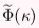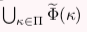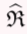Pseudo-code representing functional style

I’m preparing a scientific publication in which I want to illustrate how to implement a well-known finite automata algorithm using immutable data structures and functional programming style. I have an implementation in Scala and also in Common Lisp. If possible I’d like to illustrate the algorithm in the article without language specifics.

QUESTION: can someone suggest how to represent this algorithm in pseudo-code in a way that is easy to understand?

Usually representing code as pseudo code is imperative. And LaTeX packages have beautifully typeset symbols for assignment, looping, etc. I’m not aware of mathematical notation to represent flat-map, group-by, fixed-point, reduce, etc. I’m tempted to invent some notation, but that would be even more baggage for the reader to understand.

Leaving the code in Scala syntax, is undesirable, as there is lots of syntax for type parameters, local functions (including _ functions) , subtle scoping rules, for-comprehension, destructuring,

The implementation in Scala is given below. I have defined two functions as helpers fixedPoint which continues to call the given function, each time with the return value of the previous call, until the output value is close-enough to the input. There’s nothing surprising about fixedPoint.

def fixedPoint[V](value: V, f: V => V, cmp: (V, V) => Boolean): V = {
@tailrec
def recur(value: V): V = {
val newValue = f(value)
if (cmp(value, newValue))
value
else
recur(newValue)
}

recur(value)
}

I’ve also defined a variant of groupBy called partitionBy which returns the .values of the groupBy Map converted to a Set. This function basically returns the canonical partition of the input domain according to the partitioning function f – but with an optimization, that if the input domain contains exactly one element, there’s no reason to attempt to partition it.

def partitionBy[S,T](domain:Set[S], f:S=>T):Set[Set[S]] = {
if (domain.size == 1)
Set(domain)
else
domain.groupBy(f).values.toSet
}

The main algorithm in question is called findHopcroftPartition. It is usually explained imperatively. However, in my opinion the function understanding is elegant.

The functions Phi and phi are defined extensively in the paper, thus their terse names here. The value returned by findHopcroftPartition is the fixedPoint of a function called refine starting with a partition called Pi0 (also calculated with a call to partitionBy). Whereas refine evaluates to the given partition flat-mapped with a re-partition function called repartition. And finally repartition is just a partitionBy (cousin of groupBy) using the Phi function as the partitioning function.

In my mind, understanding what Phi does is subtle and risks being tedious to implement, but given that the reader accepts Phi, the rest is an easy to understand functional programming idiom.

Additionally, Phi itself can be elegantly expressed (in Scala anyway) as a map, reduce, groupBy, destructuring, and locally defined type aliases.

def findHopcroftPartition[L, E](dfa: Dfa[L, E]): Set[Set[State[L, E]]] = {
type STATE = State[L, E]
type EQVCLASS = Set[STATE]
type PARTITION = Set[EQVCLASS]

val Pi0 = partitionBy(dfa.F, dfa.exitValue) + dfa.Q.diff(dfa.F)

def partitionEqual(pi1    : PARTITION, pi2: PARTITION): Boolean =
pi1 == pi2

def refine(partition: PARTITION): PARTITION = {
def phi(source: STATE, label: L): EQVCLASS = {
partition.find(_.contains(source.delta(label))).get
}

def Phi(s: STATE): Set[(L, EQVCLASS)] = {
val m = for {
(eqvClass, trans) <- s.transitions.groupBy(tr => phi(s, tr.label))
label = trans.map(_.label).reduce(dfa.combineLabels)
} yield (label, eqvClass)
m.toSet
}
def repartition(eqvClass:EQVCLASS):PARTITION = {
partitionBy(eqvClass,Phi)
}
partition.flatMap(repartition)
}

fixedPoint(Pi0, refine, partitionEqual)
}

I’m tempted to say that it may be worth looking into Haskell for this. I’m no expert, but it seems to me that FP descriptions of algorithms in papers often show up as Haskell, since the language is pretty optimized for that…

1 Like

Here is what I have so far. I have defined the tilde operatoras the group-into-equivalence-classes function. And I have realized that flat-map on a set of setssimply the union operator. I don’t have a notation for fixed point. I’m pretty sure I’d be able to locate one with a bit of research or from stack-overflow.

If I define circumflex as a fixed-point operator,,then I can reduce the algorithm to the following.

I recommend to check out mu-calculus for fixed point notation: